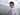# 11. 盛最多水的容器

December 04, 2019

https://leetcode-cn.com/problems/container-with-most-water/

## 解法一：

``````func maxArea(height []int) int {
var max int
for i := range height {
area := _maxArea(height[i:])
if area > max {
max = area
}
}
return max
}

func _maxArea(height []int) (max int) {
if len(height) <= 1 {
return
}
startH := height
for i, h := range height {
var area int
if h < startH {
area = i * h
} else {
area = i * startH
}
if area > max {
max = area
}
}
return
}``````

## 解法二：

``````func maxArea(height []int) int {
i := 0
j := len(height) - 1
var max int
for i < j {
var area int
if height[i] > height[j] {
area = (j - i) * height[j]
j--
} else {
area = (j - i) * height[i]
i++
}
if area > max {
max = area
}
}
return max
}``````

``area = width * height``

height 到 height[len(height) - 1] 是宽度最大的时候，此后当我们逐渐向后遍历时，宽度肯定是在逐渐减小的，因此我们要保证高度是在逐渐增大的，才有可能得到的面积比 (height, height[len(height) - 1]) 的大。